# Kerala Syllabus 10th Standard Maths Solutions Chapter 6 Coordinates

## Kerala State Syllabus 10th Standard Maths Solutions Chapter 6 Coordinates

### Coordinates Text Book Questions and Answers

Textbook Page No. 134

Question 1.
Find the following.
i. The y -coordinate of any point on the x-axis.
ii. The x -coordinate of any point on the y-axis.
iii. The coordinates of the origin
iv. The y-coordinate of any point on the line through (0,1), parallel to the x-axis.
v. The x- coordinate of any point on the line through (1,0), parallel to the y-axis.
i. y-coordinate of any point on the x-axis is zero.
ii. x-coordinate of any point on the y-axis is zero.
iii. Origin is (0, 0).
iv. y = 1
v. x =1

Kerala Coordinates Question 2.
Find the coordinates of the other three vertices of the rectangle below: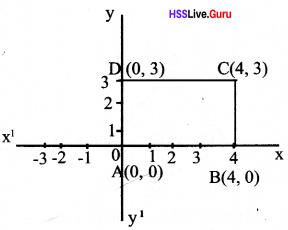A(0,0), B (4,0), D(0,3)

Class 10 Maths Chapter 6 Question 3.
In the rectangle shown below, the sides are parallel to the axes and origin is the midpoint: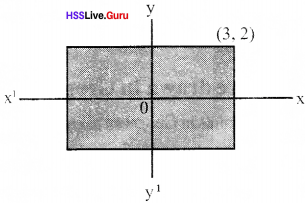What are the coordinates of the other three vertices?B (–3, 2), C(–3, -2). D (3, –2)Vipin Mahatma it Tutorial Class 10 Chapter 6 Question 4.
The triangle shown below is equilateral: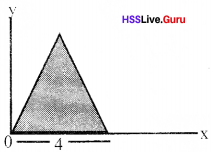Find the coordinates of its vertices.
ΔOAB is an equilateral triangle. So angles of AOCB are 30°, 60°, 90°.
∴ Its sides are in the ratio of 1: √3: 2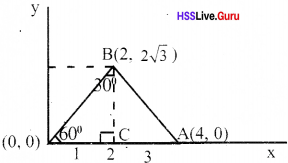OC = 2, BC = 2 √3
Hence the coordinates are B (2, 2, √3), A (4, 0), O(0, 0)

Chapter 6 Maths Class 10 Question 5.
A large trapezium made up of four equal trapeziums:Find the coordinates of the vertices of all these trapeziums. Draw this picture in GeoGebra.
In trapezium (1). Coordinates of the vertices are (0, 0), (2, 2), (4, 2), (4, 0)
In trapezium (2). Coordinates of the vertices are (4,0), (4,2), (6, 2), (8, 0)
In trapezium (3), Coordinates of the vertices are (2, 2), (4, 4), (6, 4), (6, 2)
In trapezium (4). Coordinates of the vertices are (8, 0), (6, 2), (6, 4), (8, 4)
For drawing figure in GeoGebra, Put input in the sequence of input bar as [(a, a + 1), a, 0.5,0.5].Question 6.
In the picture the centre O of the circle is the origin and A, B are points on the circle. Calculate the coordinates of A and B.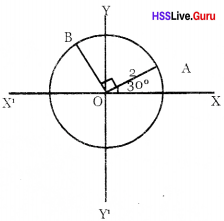As the angles are in the ratio 30 : 60: 90 the sides will be in the ratio 1: √3: 2
that is A(√3, 1), B(–1, √3)

Textbook Page No. 139

Maths Chapter 6 Class 10 Question 1.
All rectangles given below have sides parallel to the axes. Find the coordinates of the remaining vertices of each.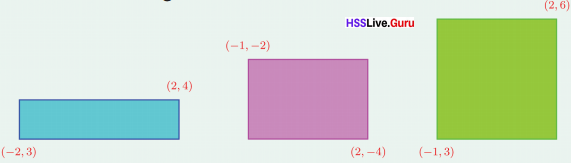Coordinates Questions Year 6 Question 2.
Without drawing coordinate axes, mark each pair of points below with left-right, top-bottom position correct. Find the other coordinates of the rectangles drawn with these as opposite vertices and sides parallel to the axes.
i. (3, 5), (7, 8)
ii. (6, 2), (5, 4)
iii. (-3, 5), (-7, 1)
iv. (-1, -2), (-5, -4)
i. If A (3, 5) and C (7, 8) then other coordinates are, B (7, 5), D (3, 8)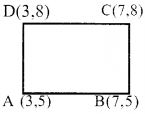ii. If B(6, 2) and D (5, 4) D<5,4)other coordinates are A (5, 2) & C (6, 4)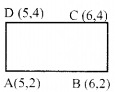iii. If A(-7, 1) and C (-3, 5) other coordinates are B(-3, 1), D (-7, 5)iv. If A(-5, -4) and C(-1, -2) other coordinates are B(-1, -4), D (-5, -2)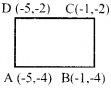Textbook Page No. 146

Question 1.
Calculate the length of the sides and diagonals of the quadrilateral on the right.Let AC, BD be the diagonals,Length of AC = Distance between the points (-3, -2) and (0, 0)Length of BD = Distance between the points (1, -2) and (-3, 1)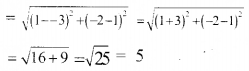Question 2.
Prove that by joining the points (2, 1), (3, 4), (-3, 6) we get a right triangle.
Let, A(2, 1), B(3, 4), C(-3, 6) be the vertices of the triangle ABC.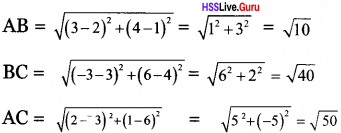Using Pythogoras theorem AC2 = AB2 + BC2
50 = (√10)2 + (√40)2 = 50
∴ We obtain a right triangle.

Question 3.
A circle of radius 10 is drawn with the origin as centre.
i. Check whether each of the points with coordinates (6, 9), (5, 9), (6, 8) is inside, outside or on the circle.
ii. Write the coordinates of 8 points on this circle.i. Distance of the point (5, 9) from the centre of the circle = $$\sqrt{25+81}=\sqrt{106}>10$$
Distance of the point (6, 9) from the centre of the circle = $$\sqrt{36+81}=\sqrt{117}>10$$
Distance of the point (6, 8) from the centre of the circle = $$\sqrt{36+64}=\sqrt{100}=10$$
(5, 9), (6, 9) are outside the circle (6, 8) is on the circle

ii. (10, 0), (0, 10), (–10, 0), (0, –10)
(6, 8), (–6, 8), (6, –8), (–6, –8)

Question 4.
Find the coordinates of the points where a circle of radius √2, centred on the point with coordinates (1, 1) cuts the axes.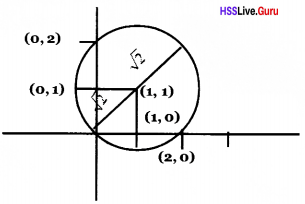It cuts at (0, 0), (2, 0) on the x-axis and at (0, 0), (0, 2) on the y-axis.

Question 5.
The coordinates of the vertices of a triangle are (1, 2), (2, 3), (3, 1), Find the coordinates of the centre of its circumcircle and the circumradius.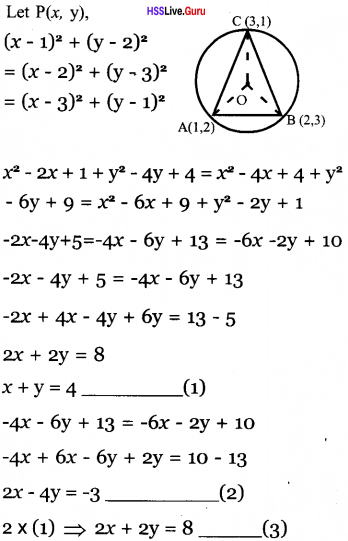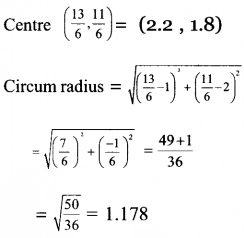### Coordinates Orukkam Questions and Answers

Worksheet 1

Question 1.
Draw x, y axis and mark the points A(0, 5), B(0, –2), C(4, 0), D(–3, 0), E(4, 5), F(–3,–2), G(4, –2)Points on x axis = – D(–3, 0), C(4, 0)
Points on y axis = A(0, 5), B(0, –2)

Question 2.
What are the points on x-axis, on y-axis?Points on x axis = – D(–3, 0), C(4, 0)
Points on y axis = A(0, 5), B(0, -2)

Question 3.
Write coordinates of two more points on AE
(1, 5), (2, 5)

Question 4.
Write the coordinates of two more points onCE.
(4, 1), (4, 2)

Worksheet 2

Question 5.
Given A(2, 3), B(5, 4), C(6, 7), D(3, 6). Find the lengths AB, BC, CD, AD.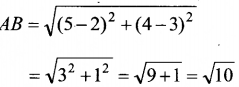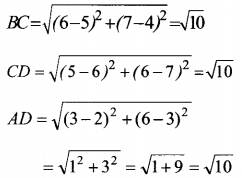Question 6.
Check whether AC = BD or not.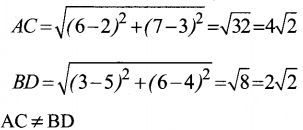Question 7.
Prove that P(4,5) the point on AC and BD.AP +PC = 4√2 = AC
∴ P (4, 5) is a point on AC .BP + PD = √2 + √2 = 2√2 = BD
∴ P (4, 5) is also a point on BD.

Question 8.
Find a point on x-axis equidistant from A and B.
Let (x, 0) be a point on the x axis which is at equal distance from A and B .
(2 – x)2 + (3 – 0)2= (5 – x)2 + (4 – 0)2
(2 – x)2 + 9 = (5 – x)2 + 16
(2 – x)2 + (5 – x)2 = 16 – 9
4 – 4x + x2 – 25 + 10x – x2 = 7
6x = 28
x = $$\frac { 28 }{ 6 }$$ = $$\frac { 14 }{ 3 }$$
∴ Point on x axis is $$\left(\frac{14}{3}, 0\right)$$

Worksheet 3

Question 9.
The sides of ABCD are parallel to the coordinate axes and A(3, 7), C(7, 9) are the opposite vertices. Write the coordinates of B and D.Question 10.
Find the lengths of AB and BC.
AB = |7 – 3| = 4 BC = |9 – 7| = 2

Question 11.
Calculate the area of the rectangle ABCD
Area of the rectangle ABC D = AB × BC
4 × 2 = 8 m2

Question 12.
If P, Q, R, S are the midpoints of the sides write the coordinates of P, Q, R, S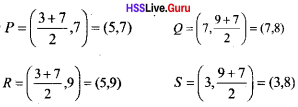Question 13.
Calculate the sides of PQRS.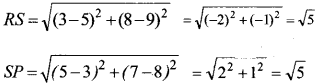Question 14.
Suggest a name suitable to PQRS
Rhombus (SQ = 4, RP = 2, diagonals are not equal, sides are equal hence it is a rhombus)

Worksheet 4

Question 15.
If A(4, 3), B(-4, 3) are two points on the line AB write two more points on this line.
A(4, 3), B(-4, 3)
Hence other points on AB = (-2, 3) (1, 3)

Question 16.
Write the coordinates of two more points on the line perpendicular to AB and passing through (4, 3)
(4, 1) (4, 5)

Question 17.
Find the length AB.Question 18.
Write the coordinates of the mid point of AB.
Coordinates of the mid point of AB = $$\left(\frac{4+-4}{2}, 3\right)=(0,3)$$

Worksheet 5

Question 19.
Without drawing coordinate axes mention the positions of A(2, 1), B(6, 1), C (6, 5) as left-right, above-belowQuestion 20.
Draw coordinate axes, mark the points and complete triangle ABC.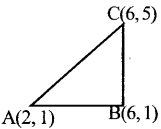Question 21.
Which side of the triangle is parallel to x-axis?
AB, parallel to x-axis.Question 22.
Which side is parallel to y-axis?
BC, parallel to y-axis.

Question 23.
Write the coordinates of the mid point of AB
Coordinates of the mid point of AB = $$\left(\frac{2+6}{2}, 1\right)=(4,1)$$

Question 24.
Write the coordinates of the midpoints of BC.
Midpoints of BC = $$\left(6, \frac{5+1}{2}\right)=(6,3)$$

Question 25.
Write the coordinates of the midpoints of AC.
Midpoints of AC = $$\left(\frac{2+6}{2}, \frac{1+5}{2}\right)=(4,3)$$

Worksheet 6

Question 26.
A (6, 0) is a point on a circle with centre (0,0). Find the radius of the circle.
Radius of the circle = 6

Question 27.
Show that B (–3, 3√3) ,C(–3, –3√3) are the points on this circle
Distance of B from origin $$\sqrt{(-3-0)^{2}+(3 \sqrt{3}-0)^{2}}$$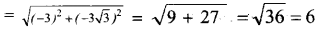Distance of C from origin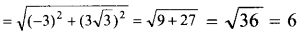B(–3, 3√3) and C(–3, –3√3) are on the circle.

### Coordinates SCERT Questions and Answers

Question 28.
Consider an equilateral triangle ABC with A (0,3) AD is the height. If G is the centroid and D is the origin, Find the coordinates of B, C, D and G. [Score: 3, Time: 6 minutes]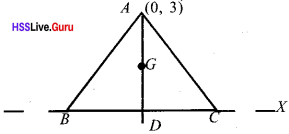D is the origin coordinates is (0, 0) (1)
G divides AD in the ratio 2 : 1
∴ Coordinates of G is (0,1) (1)
Triangle ADB is a right triangle with 30°, 60°, 90°
∴ BD = $$\frac { 3 }{ √3 }$$ = √3
∴ Coordinates of B is (–√3, 0) (1)
∴ Coordinates of C is (√3, 0) (1)

Question 29.
Find the coordinates of a point on the x -axis which is at a distance of 5 units from (4, -5). Find the coordinates on the y-axis [Score: 3, Time: 6 minutes]
Thc point on x-axis which is at a distance of 5 units from (4,-5) is (4, 0).
(x – 4)2 + 52 = 25,
∴ x = 4
Point on x axis is (4, 0) (1)
Point on y axis is (0, y).
(0 – 4)2 + (y + 5)2 = 25,
∴ y = –8, –2
The point on y -axis which is at a distance of 5 units from (4, –5) are (0, –2), (0, –8)

Question 30.
Consider a rhombus ABCD whose diago¬nals meet at origin. The length of diagonals are 8 units and 6 units. Find the coordinates of the vertices. [Score: 4, Time: 4 minutes]
Find the coordinates of the vertices by drawing the figure.
(3, 0), (–3, 0), (0, 4), (0, –4)Question 31.
Find the coordinates of the vertices of a square of side 10 units whose diagonals meet at origin. [Score: 4, Time: 4 minutes]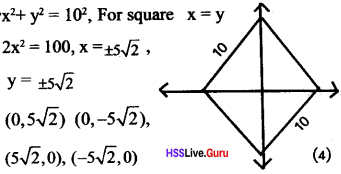Question 32.
Consider a regular hexagon of side 6 units whose diagonals meet at the origin. Find the coordinates of the vertices. [Score: 5, Time: 8 minutes]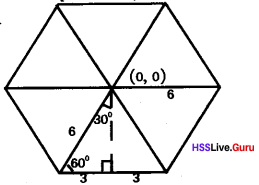(6, 0) (0, 6)
Find the coordinates of the vertices using the concept of right-angle triangles 30°, 60°, 90° (1)
(3, 3√3), (–3, 3√3), (3, –3√3), (–3, –3√3) (3)

Question 33.
Find the coordinates of other points.. [Score: 4, Time: 8 minutes]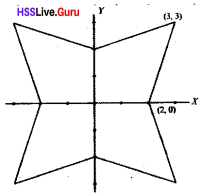Points are (–2, 0), (0,2), (0, –2) (2)
Points are (3, –3), (–3, –3), (–3,3) (2)

Question 34.
Find the coordinates of the fourth vertices of the parallelogram. Find the length of the sides of the parallelogram. Write the length of diagonals. [Score: 5, Time: 8 minutes]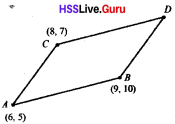The difference of x-coordinate of the points A, C = 2
The difference of x-coordinate of the points B, D = 2
Hence the x-coordinate of the point x = 11
The difference of y-coordinate of the points A, C = 7 – 5 = 2
The difference of y-coordinate of the points B, D = 2
Hence the y-coordinate of the point x = 10 + 2 = 12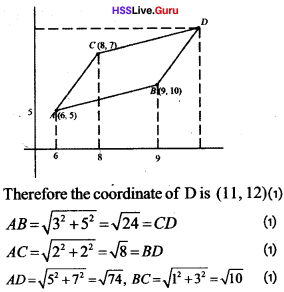Question 35.
Consider a rhombus of side 10 units whose diagonals are coordinate axis. If an angle is 120°, Find the coordinates of the other vertices. [Score: 4, Time: 6 minutes]
A rhombus is formed by joining two equilateral triangles. Using the right triangle of 30°, 60°, 90°, find the diagonals as 10,10√3 units. Hence the coordinates of the vertices of the rhombus are(5, 0) (-5, 0)(0,5√3), (0,-5√3). (4)

Question 36.
Find the coordinates ofthe points on x-axis which are equidistant from the points (-5, 8) and (6, -4).[Score: 4,Time: 8 minutes]
The y-coordinate of points on x-axis will be zero. Hence assume that a point on x-axis is (x, 0)
Distance between (x, 0) and (-5, 8) = $$\sqrt{(x-5)^{2}+8^{2}}$$
Distancebetween (x, 0) and (6, 4) = $$\sqrt{(x-6)^{2}+(-4)^{2}}$$
(x + 5)2 + 82 = (x – 6)2 + (–4)2
x2 + 10x + 25 + 64 = x2 – 12x + 36 + 16
22x = 36 + 16 – 25 – 64 = 52 – 89 = –37
x = $$\frac {–37 }{ 22 }$$
Coordinate $$\left(\frac{-37}{22}, 0\right)$$ (4)

Question 37.
Prove that A (4,5), B (4,2), C(8,2) represents the vertices of a right angled triangle. Find the coordinate of the circumcentre. What is the radius of the circumcircle? [Score: 4, Time: 8 minutes]
Distance between (4, 5) and (4, 2) = 5 – 2 = 3
Distance between (4, 2) and (8, 2) = 8 – 4 = 4
Distance between (4, 5) and (8, 2)3, 4, 5 are sides of a right angled triangle,
since 32 + 42 = 52 (1)
The coordinates of circumcentre is (6 ,3, 5) (1)
Radius of circumcircle = 2.5 unit (1)Question 38.
P is a point on the perpendicular bisector of the line joining (2, 5) and (6, 5). If the x coordinate and y coordinate of P are equal write the coordinate of P. [Score: 5, Time: 8 minutes]
Understanding the concept that the distance of a point on the perpendicular bisector is equidistant from the endpoints. (1)
Assume that the coordinate of P is (a, a)
Then; Distance between (a, a) and (2, 5)Coordinate of P (4, 4) (1)

Question 39.
Write the coordinate of the centre of a circle passing through the points (9, 3), (7,-1) (1,-1). Find the radius of the circle. [Score: 5, Time: 8 minutes]
Assume that (x, y) is centre of the circle Distance between (9,3) and (x,y)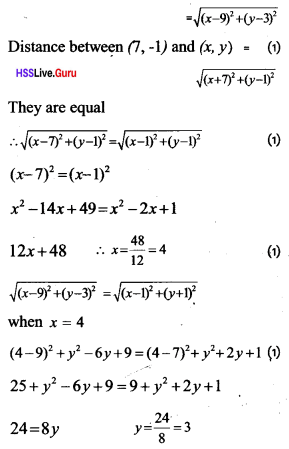The coordinate of the centre of the circle is (4, 3)
∴ Radius = Distance between (4, 3) and (1,-1)
= $$\sqrt{(4-1)^{2}+(3+1)^{2}}=\sqrt{3^{2}+4^{2}}=5$$ (1)

Question 40.
If (x, y) be apoint equidistant from the points (7, 5), (4, 3).then show that 6x + 4y = 49 [Score: 4, Time: 7 minutes]
Distance between (x, y) and (7, 5)
$$\sqrt{(x-7)^{2}+(y-5)^{2}}$$ (1)
Distancebetween (x, y) and (4, 3)
$$\sqrt{(x-4)^{2}+(y-3)^{2}}$$ (1)
They are equal
(x – 7)2 + (y – 5)2 = (x – 4)2 + (y-3)2
by solving
6x + 4y = 49 (2)

Question 41.
Three vertices of a square are given. If the fourth vertex is (2, P) find the ratio of P. Find the area of the square. [Score: 4, Time: 7 minutes]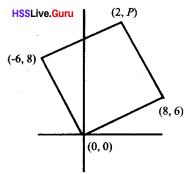Question 42.
In the figure if P (36, 48), then find the coordinates of A, B, M [Score: 4, Time: 7 minutes]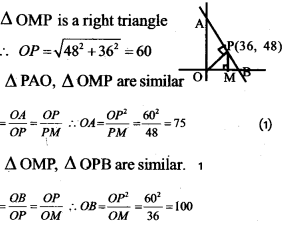Coordinates of M (36, 0) (1)
Coordinates of A (0, 75) (1)
Coordinates of B (100, 0) (1)

### Coordinates Exam Oriented Questions and Answers

Short Answer Type Questions (Score 2)

Question 43.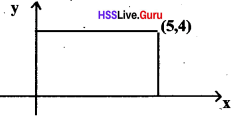Find the coordinates of die other three verti¬ces of the rectangle in the figure.The coordinates of are (0, 0), ( 5, 0), (5, 4)

Question 44.
Check whether the points P(0, 7), Q(5, 12), R(-10, -3) lie on a single line.∴ The three points are on the same line.

Question 45.
The origin in the diagram is o. The endpoints of the diameter of the circle are A and B. Find the coordinates of P. OQ is perpendicular to OP. Find out the coordinates of Q.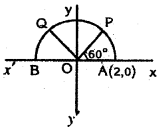30: 60: 90 = 1: √3: 2
Coordinates of p is (√3, 1)
The Coordinates of Q = (–1, √3)

Question 46.
ABCDEF is a regular hexagon. Of these points C and D are on the number line. C is three unit to the left of zero and D is 5 unit to the right. What is the length of the side EF? What is the measure of an angle of the regular hexagon?
CD = |5– –3| = 8
∴ CD = 8 unit
EF = 8 unit
Angle of a regular hexagon = 720 ÷ 6 = 120°Question 47.
A circle is drawn with centre at (-1, 0) and radius 5 units in a coordinate system. What are the coordinates of the points at which it cuts the x-axis and the points where it cuts the y-axis?Question 48.
A circle is drawn with centre at (0, 0) and radius 6 units in a cooredinate system. What are the coordinates of the points which it cuts the x-axis? And the points where it cuts the y-axis?
x axis (6,0) and (–6,0)
y axis (0, 6 ) and (0, –6)

Short Answer Type Questions (Score 3)

Question 49.
Find the length of the sides of the rectangle given in the figure.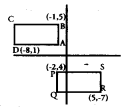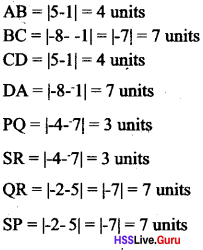Question 50.
A small rectangle is cut from the centre of the big for rectangle. Based on the perpendiculars drawn at the centre of the big rectangle, find out the coordinates of the vertices of the small rectangle cut.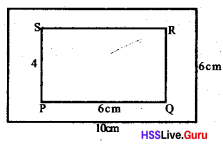P(–3, –2), Q(3, –2), R(3, 2), S(–3, 2)

Question 51.
Each side of the square OPQR given in the diagram is 6 unit. Write the coordinates of the vertices of the square.P(6, 0), Q(6, 6), R(0, 6), O(0, 0)

Question 52.
a. In the figure ABCD is a square. If A is (2, 0) then find the coordinates of B,C, D. Also find the coordinates of the centre.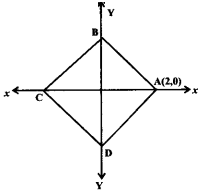b. Classify the points (5, 0), (0, –2), (–2, 0), (2, 3), (10, 9), (0, 8) and (6, 0) according to:
i. Points on the x-axis
ii. Points on the y-axis
iii. Does not belong to the axis
a. B(0, 2), C(–2, 0), D(0, –2) Coordinate of vertex (0,0)

b, i. (5, 0), (–2, 0), (6, 0)
ii. (0, –2), (0, 8)
iii. (2, 3), (10, 9)

Question 53.
Selecting the given pair of coordinates given below, mark the opposite corners of a rectangle whose sides are parallel to the axis. Measure the lengths of the sides. Find out the coordinates of other points.
a (4, –4), (–4, 4)
b. (2, 1), (6, 5)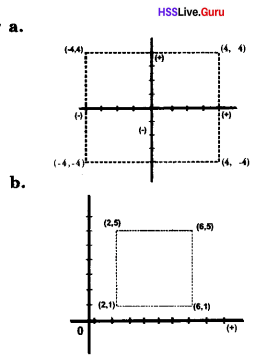Long Answer Type Questions (Score 4)

Question 54.
a. Write the coordinates of the points of intersection of the circle with the axis, where circle having radius 10cm and centre at the origin.
b. Write the co-ordinates of a point which are not on the circle.
a. (10, 0), (0, 10); (–10, 0), (0, –10)
b. (9, 6); ie, all the point which satisfies.
x2 + y2= 102 are on the circle, others are inside or outside the circle

Question 55.
Calculate the length of sides of quadrilateral.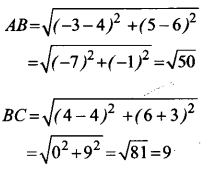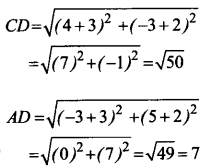Question 56.
P is a point inside the rectangle ABCD. Prove that PA2 + PC2 = PB2 + PD2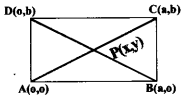Take AB as x axis and AD as y axis, a and b are the sides of the rectangle. The coordinates of the points are marked on the picture.
PA2 + PC2 = x2 + y2 + (x – a)2 + (y – b)2
PB2 + PD2 = (x – a)2 + y2 + x2 + (y – b)2
PA2 + PC2 = PB2 + PD2

Long Answer Type Questions (Score 5)

Question 57.
In ΔABC, drawn above the x axis with ∠A=90° the coordinates of A (2,0) and that of B (8,0). The length of AC is 5 units and the area of the triangle is 12 square units. Draw a rough sketch of coordinate axis and the triangle. Find the coordinates of C.
$$\frac { 1 }{ 2 }$$ × AB × h = 12
$$\frac { 1 }{ 2 }$$ × 6 × h = 12
h = 4 units
AC = 5
PC = 4 then
AP = 3
∴ OP = 5, PC = 4, C(5,4)Question 58.
Find out the coordinates for the other vertices of the rectangle ABCD in the diagram. If the unit used to measure the length is 3/4cm, Find out the area of the rectangle ABCD.C(6, 2); D(2, 2)
Length of the rectangle = 4 unit,
Unit= 3/4 cm,
Length = 4 × 3/4 = 3cm
Breadth = 2 × 3/4 = 3/2 = 1 1/2cm
Area = 3 × 1.5 = 4.5 cm2

Question 59.
a. From the points given below, find the pair which are on a line parallel to the x – axis and the pair which are on a line parallel to the y-axis.
A(4, 3), B(3, 5), C (–6, 3),
D(3, –2), E(5, 4)
b. Find the distance between the points given below
i. (–3, 2) and (4, 2)
ii. (5, 8) and (6, 9)
a. Parallel to the x-axis A(4, 3) and C(–6, 3)Parallel to the y-axis B(3, 5) and D(3, –2)
b. i. Distance = |–3 –4| = 7### Coordinates Memory Map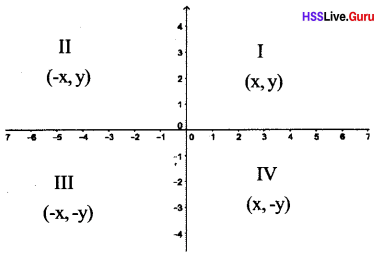Horizontal line is x-axis and vertical line is y-axis.

Point of intersection of the x- axis and y axis is called the origin O. Numbers which are above and to the right side of the origin O will be always positive while numbers on left and below the origin will be negative.

All points on the x – axis have y – coordinates zero and all points on y-axis have x- coordinates zero.